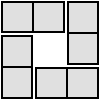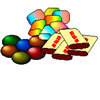#### You may also like### Domino Square

Use the 'double-3 down' dominoes to make a square so that each side has eight dots.### 4 Dom

Use these four dominoes to make a square that has the same number of dots on each side.### The Puzzling Sweet Shop

There were chews for 2p, mini eggs for 3p, Chocko bars for 5p and lollypops for 7p in the sweet shop. What could each of the children buy with their money?

# Plenty of Pens

##### Age 7 to 11Challenge Level

Adrian has worked out this problem very efficiently:

No of pencils $= x$
No of pens $= 4x$

Spent on pencils $= 10x$
Spent on pens $= 4x \times15 = 60x$
Total spent $= 10x + 60x = 70x$

$70x = 250 - 40$
$70x = 210$
$x = 210 / 70 = 3$

Therefore no of pencils $= 3$
No of pens $= 3 \times4 = 12$

Pupils at Moorfield Juniors, including Emma, approached it slightly differently:

We worked this out by taking 40p from £2.50 which is 2.10 then using trial and error.
It was easy because there aren't many low numbers in the 4 times table.
The answer is 12 pens which equals £1.80. 12 divided by 4 = 3 so there are 3 pencils which equals 30p.
£1.80+30p= £2.10.